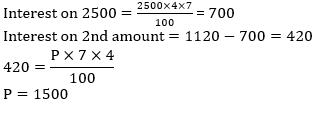Courses

# Test: Mathematics-1

## 20 Questions MCQ Test CTET ( Central Teacher Eligibility Test ) Mock Test Series | Test: Mathematics-1

Description
This mock test of Test: Mathematics-1 for Teaching helps you for every Teaching entrance exam. This contains 20 Multiple Choice Questions for Teaching Test: Mathematics-1 (mcq) to study with solutions a complete question bank. The solved questions answers in this Test: Mathematics-1 quiz give you a good mix of easy questions and tough questions. Teaching students definitely take this Test: Mathematics-1 exercise for a better result in the exam. You can find other Test: Mathematics-1 extra questions, long questions & short questions for Teaching on EduRev as well by searching above.
QUESTION: 1

### In a classroom there are certain number of benches. If 6 students are made to sit on a bench, then to accommodate all of them, one more bench is needed. However, if 7 students are made to sit on a bench, then, after accommodating all of them, space for 5 students is left. What is the total number of students in the class?

Solution: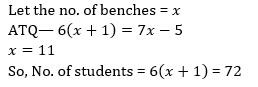QUESTION: 2

### The average monthly salary of the workers in a workshop is Rs. 8,500. If the average monthly salary of 7 technicians is Rs. 10,000 and the average monthly salary of the rest is Rs. 7,800, then the total number of workers in the workshop is

Solution: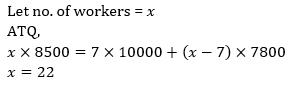QUESTION: 3

### There were 35 students in a hostel. If the number of students increases by 7, the expenses of mess increase by Rs. 42 per day while the average expenditure per head diminishes by 1. Find the original expenditure of the mess.

Solution: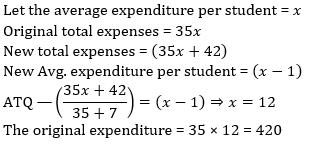QUESTION: 4

A man is 50 years old. His brother is 7 years older than him and his sister is 12 years younger than his brother. When his sister was 15 years old, then the men’s age was :

Solution: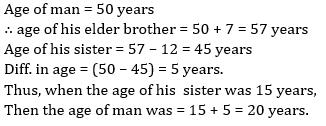QUESTION: 5

A man walked diagonally across a square plot. Approximately what was the percent distance saved by not walking along the edges?

Solution: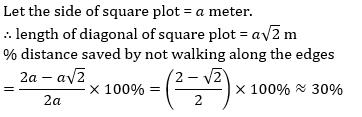QUESTION: 6

The ratio of the number of boys to that of girls in a school is 4: 1. If 75% of boys and 70% of the girls are scholarship holders, then the percentage of students who do not get scholarship is

Solution: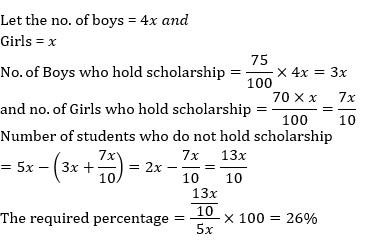QUESTION: 7

A tradesman gives 4% discount on the marked price and gives 1 article free for buying every 15 articles and thus gains 35%. The marked price is increased above the cost price by.

Solution: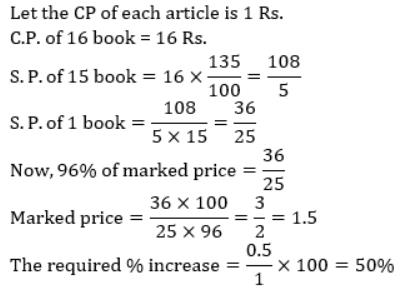QUESTION: 8

A certain amount earns simple interest of Rs. 1750 after 7 years. Had the interest per annum been 2% more, how much more interest would it have earned?

Solution: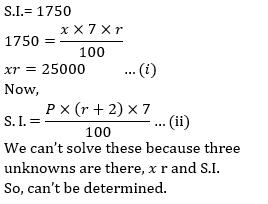QUESTION: 9

Reena borrowed Rs. 6,450 at 5 percent simple interest repayable in 4 equal installments. What will be the annual installment payable by him?

Solution: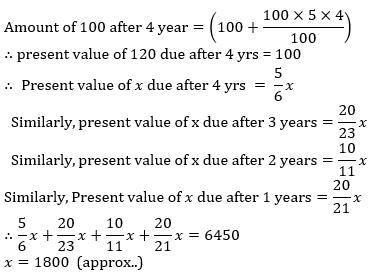QUESTION: 10

Mr. X invested an amount for 2 years at 15% per annum at simple interest. Had the interest been compounded, he would have earned Rs. 450/- more as interest. What was the amount invested?

Solution: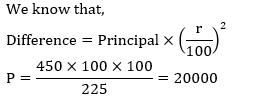QUESTION: 11

In a business, Abhiram and Chiru invested amounts in the ratio 2: 1, whereas the ratio between amounts invested by Abhiram and Bikash was 3: 2. If Rs. 1,57,300 was their profit, how much amount did Bikash receive?

Solution: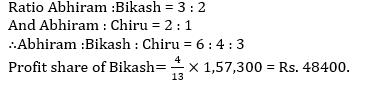QUESTION: 12

Instead of dividing of Rs. 117 among P, Q, R in the ratio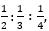, it was divided in the ratio 2 : 3: 4 by mistake. Who gained in this transaction?

Solution: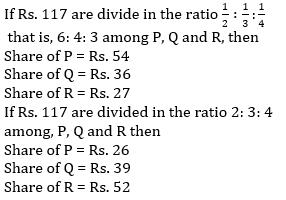QUESTION: 13

An Insurance employer reduces the number of his employees in the ratio 9: 8 and increases their wages in the ratio 14: 15. If the original wage bill was Rs. 18,900, find the ratio of decreased wage and original wage.

Solution: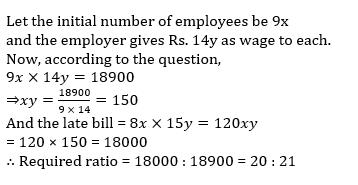QUESTION: 14

The number obtained by interchanging the digits of a two-digit number is less than the original number by 63. If the sum of the digits of the number is 11, what is the original number?

Solution: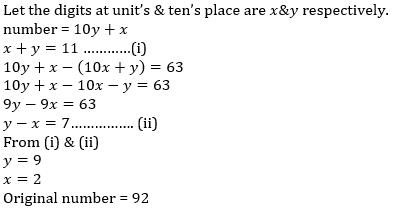QUESTION: 15

The respective ratio between the present ages of Roshan and Rakesh is 6: 11. Four years ago, the ratio of the ages was 1: 2 respectively. What will be Rakesh’s age after five years?

Solution: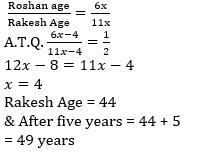QUESTION: 16

The numbers 11284 and 7655, when divided by a certain number of three digits, leave the same remainder. Find that number of three digits.

Solution: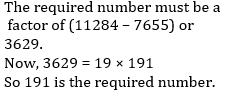QUESTION: 17

Four Iron metal rods of lengths 78 cm, 104 cm, 117 cm, and 169 cm are to be cut into parts of equal length. Each part must be as long as possible. What is the maximum number of pieces that can be cut?

Solution:

Since each Iron rod must be cut into parts of equal length and
each part must be as long as possible, so HCF should be
taken.
HCF of 78, 104, 117 and 169 = 13.
No. of parts from 78cm. rod = 78 /13=6
No. of parts from 104 cm. rod =104/13=8
No. of parts from 117 cm. rod =117/ 13=9
No. of parts from 169 cm. rod =169 /13=13
Maximum no. of pieces = 6 + 8 + 9 + 13 = 36

QUESTION: 18

A person lent Rs. 5,000 partly at the rate of 4 percent and partly at the rate of 5 percent per annum, at simple interest for purchased a wristwatch. The total interest after 2 years is Rs. 440. The sum of money lent at each of the above rates is to be divided in the ratio:

Solution: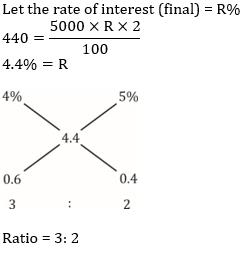QUESTION: 19

Simple interest on a certain amount is 9/16 of the principal. If the numbers representing the rate of interest in percent and time in years be equal, then time, for which the principal is lent out, is:

Solution: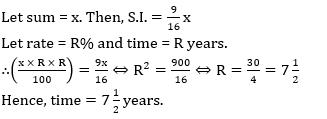QUESTION: 20

Anubhav lends Rs. 2500 to Bose and a certain sum to Chandra at the same time at 7% annual simple interest. If after 4 years Anubhav received interest of Rs. 1120 from Bose and Chandra, the sum lent to Chandra is

Solution: